# Math Practice Topic: Congruent And Similar Triangles

 Description: Recognize congruent and similar triangles. Adaptive Learning Progression: Number of problems increases. Start using MathScore for free

## Sample Levels (out of 3)

Determine whether the following sets of triangles are congruent, similar, or neither.

 1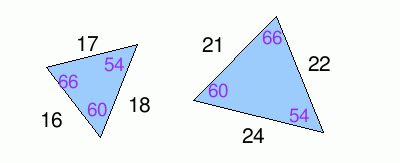Congruent        Similar        Neither 2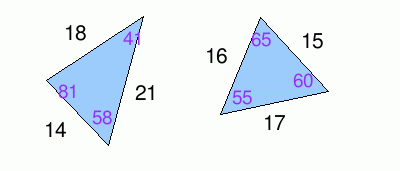Congruent        Similar        Neither

Determine whether the following sets of triangles are congruent, similar, or neither.

 1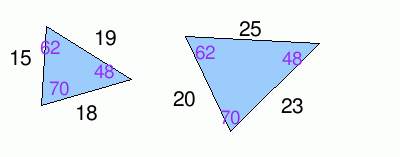Congruent        Similar        Neither 2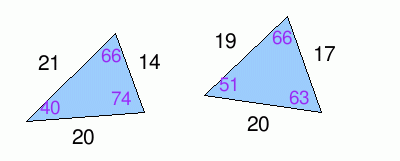Congruent        Similar        Neither 3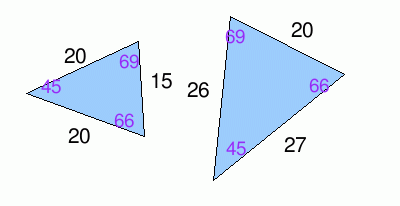Congruent        Similar        Neither 4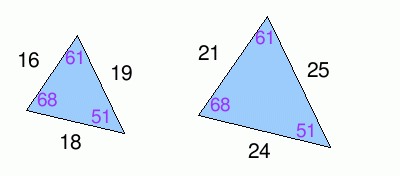Congruent        Similar        Neither 5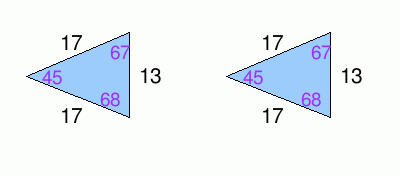Congruent        Similar        Neither 6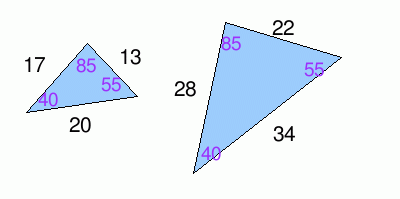Congruent        Similar        Neither 7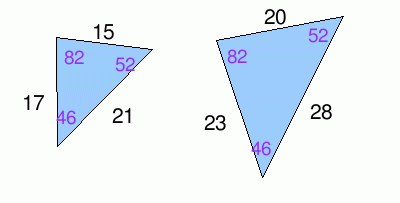Congruent        Similar        Neither 8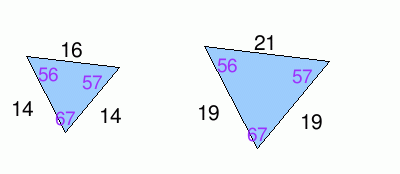Congruent        Similar        Neither